# Bihar Board Class 10th Maths Solutions Chapter 4 Quadratic Equations Ex 4.3

Bihar Board Class 10th Maths Solutions Chapter 4 Quadratic Equations Ex 4.3 Textbook Questions and Answers.

## BSEB Bihar Board Class 10th Maths Solutions Chapter 4 Quadratic Equations Ex 4.3

Question 1.
Find the roots of the following quadratic equations, if they exist by, the method of completing the square:
(i) 2x2 – 7x + 3 = 0
(ii) 2x2 + x – 4 = 0
(iii) 4x2 + 4$$\sqrt{3}$$x + 3 = 0
(iv) 2x2 + x + 4 = 0
Solution:
(i) The equation 2x2 – 7x + 3 is the same as∴ 2x2 – 7x + 3 = 0∴ The roots of the given equation are 3 and $$\frac{1}{2}$$.

(ii) We have:
2x2 + x – 4 = 0∴ The roots of the given equation are
$$\frac{-1+\sqrt{33}}{4}$$ and $$\frac{-1-\sqrt{33}}{4}$$

(iii) We have:
4x2 + 4$$\sqrt{3}$$x + 3 = 0
or (2x)2 + 2 × (2x) × $$\sqrt{3}$$ + ($$\sqrt{3}$$)2 – ($$\sqrt{3}$$)2 + 3 = 0
or (2x + 3)2 – 3 + 3 = 0
or (2x + $$\sqrt{3}$$)2 = 0
i.e; x = – $$\frac{\sqrt{3}}{2}$$ and – $$\frac{\sqrt{3}}{2}$$

(iv) We have,
2x2 + x + 4 = 0
or x2 + $$\frac{1}{2}$$x + 2 = 0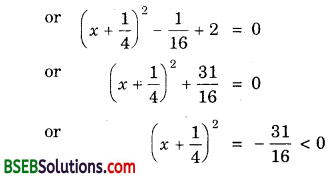But (x + $$\frac{1}{4}$$)2 cannot be negative for any real value of x.
So, there is no real value of x satisfying the given equation. Therefore, the given equation has no real roots.Question 2.
Find the roots of the quadratic equations given in Q. 1 above by applying the quadratic formula.
Solution:
(i) The given equation is 2x2 – 7x + 3 = 0.
Here, a = 2, b = – 7 and c = 3.
∴ D = b2 – 4ac = (- 7)2 – 4 × 2 × 3 = 49 – 24 = 25 > 0
So, the given equation has real roots given by∴ The roots of the given equation are 3 and $$\frac{1}{2}$$

(ii) The given equation is 2x2 + x – 4 = 0
Here, a = 2, b = 1 and c = – 4.
∴ D = b2 – 4ac = (1)2 – 4 × 2 × (- 4) = 1 + 32 = 33 > 0
So, the given equation has real roots given by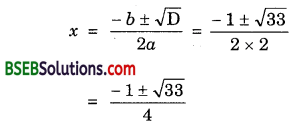∴ The roots of the given equation are
$$\frac{-1+\sqrt{33}}{4}$$ and $$\frac{-1-\sqrt{33}}{4}$$

(iii) The given equation is 4x2 + 4$$\sqrt{3}$$x + 3 = 0
Here, a = 4, b = 4$$\sqrt{3}$$ and c = 3.
∴ D = b2 – 4ac = (4$$\sqrt{3}$$)2 – 4 × 4 × 3 = 48 – 48 = 0
So, the given equation has real equal roots given by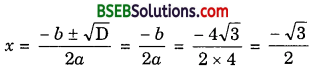∴ The equal roots of the given equation are
$$\frac{- \sqrt{3}}{2}$$ and $$\frac{- \sqrt{3}}{2}$$

(iv) The given equation is 2x2 + x + 4 = 0
Here, a = 2, b = 1 and c = 4.
∴ D = b2 – 4ac = (1)2 – 4 × 2 × 4 = 1 – 32 = – 31 < 0
So, the given equation has no real roots.
[Sometimes, it is easier to use quadratic formula method as it is a straight forward method.]

Question 3.
Find the roots of the following equations:
(i) x – $$\frac{1}{x}$$ = 3, x ≠ 0
(ii) $$\frac{1}{x+4}$$ – $$\frac{1}{x+7}$$ = $$\frac{11}{30}$$, x ≠ – 4, 7
Solution:
(i) The given equation is x – $$\frac{1}{x}$$ = 3, x ≠ 0.
So, x2 – 3x – 1 = 0
Here, a = 1, b = – 3 and c = – 1.
∴ D = b2 – 4ac = (- 3)2 – 4(1)(- 1) = 9 + 4 = 13 > 0
So, the given equation has real roots given by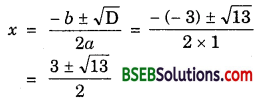∴ The roots of the given equations are
$$\frac{3+\sqrt{13}}{2}$$ and $$\frac{3-\sqrt{13}}{2}$$

(ii) The given equation is $$\frac{1}{x+4}$$ – $$\frac{1}{x-7}$$ = $$\frac{11}{30}$$, x ≠ – 4, 7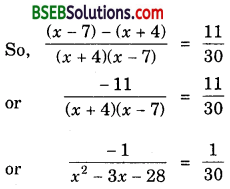or – 30 = x2 – 3x – 28
or x2 – 3x + 2 = 0
or (x – 1)(x – 2) = 0
i.e; x – 1 = 0 or x – 2 = 0
⇒ x = 1 or x = 2
Thus, 1 and 2 are the roots of the given equation.Question 4.
The sum of the reciprocals of Rehman’s ages (in years) 3 years ago and 5 years from now is $$\frac{1}{3}$$. Find his present age.
Solution:
Let Rehman’s present age be x years
As per question, we have:
$$\frac{1}{x-3}$$ + $$\frac{1}{x+5}$$ = $$\frac{1}{3}$$
or 3(x + 5) + 3(x – 3) = (x – 3)(x + 5)
or 3x + 15 + 3x – 9 = x2 + 2x – 15
or x2 – 4x – 21 = 0
or (x – 7)(x + 3) = 0 i.e., x = 7 or x = – 3
x = 7 [Since age can never be negative]
Thus, Rehman’s present age is 7 years.

Question 5.
In a class test, the sum of Shefali’s marks in Mathematics and English is 30. Had she got 2 marks more in Mathematics and 3 marks less in English, the
product of their marks would have been 210. Find her marks in the two subjects.
Solution:
Let Shefali’s marks in Mathematics be x. Then, her marks in English will be (30 – x).
As per condition of the problem:
(x + 2) × [(30 – x) – 3] = 210
or (x + 2)(27 – x) = 210
or 27x – x2 + 54 – 2x = 210
or x2 – 25x + 156 = 0
or x2 – 12x – 13x + 156 = 0
or x(x – 12) – 13(x – 12) = 0
or (x – 12)(x – 13) = 0
i.e., x = 12
or x = 13
∴ Shefali’s marks in Mathematics and English are 12 and 18, respectively on her marks in Mathematics and English are 12 and 18, respectively.

Question 6.
The diagonal of a rectangular field is 60 metres more than the shorter side. If the longer side is 30 metres more than the shorter side, find the sides of the field.
Solution:
Let in the rectangular field ABCD, BC = x metres.
Then AC = (x + 60) mctrcs and AB (x + 30) metres.
By Pythagoras Theorem, we have: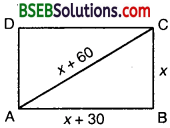AC2 = BC2 + AB2
So, (x + 60)2 = x2 + (x + 30)2
or x2 + 120x + 3600 = x2 + x2 + 60x + 900
or x2 – 60x – 2700 = 0
or x2 – 90x + 30x – 2700 = 0
or x(x – 90) + 30(x – 90) = 0
i.e; x = – 30 or x = 90
∴ x = 90 [∵ Side of a rectangle can never be negative]
Hence, the sides of the field are 120 m and 90 m.Question 7.
The difference of squares of two numbers is 180. The square of the smaller number is 8 times the larger number. Find the two numbers.
Solution:
Let the larger number be x. Then,
Square of the smaller number = 8x
Also, square of the larger number = x2
It is given that the difference of the squares of the numbers is 180.
∴ x2 – 8x = 180
or x2 – 8x – 180 = 0
or x2 – 18x + 10x – 180 = 0
or x(x – 18) + 10(x – 18) = 0
or (x – 18)(x + 10) = 0
i.e; x – 18 = 0 or x + 10 = 0
i.e; x = 18 or x = – 10

Case I:
When x = 18.
In this case, we have:
Square of the smaller number = 8x = 8 × 18 = 144
∴ Smaller number = ± 12
Thus, the numbers are 18, 12 or 18, – 12.

Case II:
When x = – 10
In this case, we have:
Square of the smaller number = 8x = 8 × – 10 = – 80
But, square of a number is always positive.
∴ x = – 10 is not possible.
Hence, the numbers are 18, 12 or 18, – 12.

Question 8.
A train travels 360 km at a uniform speed. If the speed had been 5 km/h more, it would have taken 1 hour less for the same journey. Find the speed of the train.
Solution:
Let x km/h be the uniform speed of the train.
Then, time taken to cover 360 km when the speed is increased by 5 km/hr = $$\frac{360}{x}$$ hours.
It is given that the time to cover 360 km is reduced by 1 hour.
∴ $$\frac{360}{x}$$ – $$\frac{360}{x+5}$$ = 1
or 360(x + 5) – 360x = x(x + 5)
or 360x + 1800 – 360x = x2 + 5x
or x2 + 5x – 1800 = 0
or x2 + 45x – 40x – 1800 = 0
or x(x + 45) – 40(x + 45) = 0
or (x – 40)(x + 45) = 0
i.e., x – 40 = 0 or x + 45 = 0
i.e., x = 40 or x = – 45
But speed cannot be negative. Therefore, x = 40. Hence, the original speed of the train is 40 km/h.Question 9.
Two water taps together can fui a tank in 9$$\frac{3}{8}$$ hours. The tap of larger diameter takes 10 hours less than the smaller one to fill the tank separately.
Find the time in which each tap can separately fill the tank.
Solution:
Let the smaller tap takes x hours to fill the tank. So, the larger tap will take (x – 10) hours to fill the tank.
∴ Portion of the tank filled by the larger tap in one hour
= $$\frac{1}{x-10}$$
So, Portion of the tank filled by the larger tap in 9$$\frac{3}{8}$$ hours, i.e., $$\frac{75}{8}$$ hours = $$\frac{1}{x-10}$$ × $$\frac{75}{8}$$
Similarly, portion of the tank filled by the smaller tap in $$\frac{75}{8}$$ hours = $$\frac{1}{x}$$ × $$\frac{75}{8}$$
Since it is given that the tank is completely filled in $$\frac{75}{8}$$ hours, thereforeor 150x – 750 = 8x2 – 80x
or 8x2 – 230x + 750 = 0
or 4x2 – 115x + 375 = 0
or 4x2 – 100x – 15x + 375 = 0
or 4x(x – 25) – 15(x – 25) = 0
or (x – 25)(4x – 15) = 0
i.e; x – 25 = 0 or 4x – 15 = 0
i.e; x = 25 or $$\frac{15}{4}$$
∴ x = 25, as x = $$\frac{15}{4}$$ is inadmissible ($$\frac{15}{4}$$ < 10)
Hence, the larger tap fills the tank in 15 hours and the smaller tap takes 25 hours to fill the tank.

Question 10.
An express train takes 1 hour less than a passenger train to travel 132 km between Mysore and Bangalore (without taking into consideration the time they stop at intermediate stations).
If the average speed of the express train is 11 km/h more than that of the passenger train, find the average speed of the two trains.
Solution:
Let the speed of the express train be x km/h.
Then, the speed of the passenger train is (x – 11) km/h
Total distance to be covered = 132 km
Time taken by express train = $$\frac{132}{x}$$ hours
and tame taken by the passenger train = $$\frac{132}{x-11}$$ hours
By the given condition, $$\frac{132}{x-11}$$ – $$\frac{132}{x}$$ = 1
or 132x – 132x + 1452 = x2 – 11x
or x2 – 11x – 1452 = 0
or x2 – 44x + 33x – 1452 = 0
or x(x – 44) + 33(x – 44) = 0
or (x – 44)(x + 33) = 0
i.e; x = 44 or x = – 33
But speed cannot be negative. Therefore, x = 44
∴ Speed of express train = 44 km/hr
and speed of passenger train = (44 – 11) km/h
= 33 km/hQuestion 11.
Sum of the areas of two squares is 468 m2. If the difference of their perimeters is 24 m, find the sides of the two squares.
Solution:
Let the sides of the squares be x and y metres (x > y).
According to question:
x2 + y2 = 468 ……………… (1)
and 4x – 4y = 24
i.e., x – y = 6 …………… (2)
Putting x = y + 6 in (1), we get
(y + 6)2 + y2 = 468
or 2y2 + 12y + 36 – 468 = 0
or y2 + 6y – 216 = 0
or y2 + 18y – 12y – 216 = 0
or y(y + 18) – 12(y + 18) = 0
or (y + 18)(y – 12) = 0
y = – 18 or y = 12
But side cannot be negative. Therefore, y = 12.
∴ x = y + 6 = 12 + 6 = 18
∴ The sides of the squares are 18 m and 12 m.